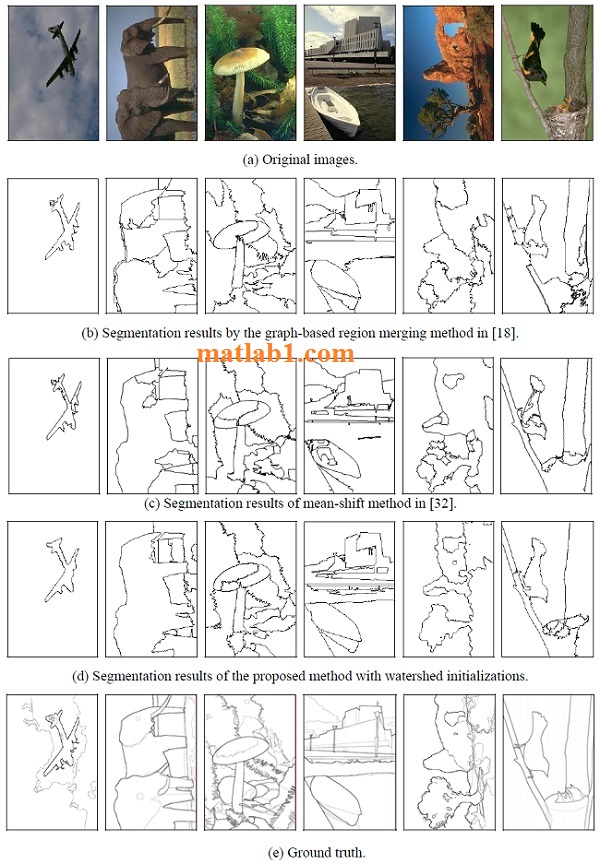# Automatic Image Segmentation by Dynamic Region Merging

\$29

## Description

In this project, we proposed a novel method for segmenting an image into distinct components. The proposed algorithm is implemented in a region merging style. We defined a merging predicate P for the evidence of a merging between two neighboring regions. This predicate was defined by the sequential probability ratio test (SPRT) and the maximum likelihood criterion. A dynamic region merging (DRM) was then presented to automatically group the initially over-segmented many small regions. Although the merged regions are chosen locally in each merge stage, some global properties are kept in the final segmentations. For the computational efficiency, we introduced an accelerated algorithm by using the data structure of region adjacency graph (RAG) and nearest neighbor graph (NNG). Experiments on natural images showed the efficiency of the proposed algorithm. There are several potential extensions to this work, such as the introduction of global refinement and user interaction, etc. Those will be further investigated in our future work.Segmentation results by different methods. The first row shows the original images. The second row shows the results by the graph-based image segmentation method . Parameter settings ([σ , K, min]) for images (from the left to the right) are (0.5, 1200, 400), (0.5, 800, 200), (0.5, 400, 200), (0.5, 1500, 700), (0.5, 800, 300) and (0.5, 500, 200), respectively. The third row shows the results by the mean-shift method , with the spatial bandwidth and color bandwidth being set as (14, 13), (13, 12), (18, 17), (14, 13), (14, 13) and (12, 11), respectively. The forth row shows the proposed method. The values of λ1 are 0.6, 0.5, 2, 1.1, 1.1 and 0.9, respectively. The fifth row shows probability-of-boundary images of the human segmentation, where the darker boundaries indicate more subjects marked a boundary .

https://matlab1.com/blood-vessels-segmentation/

Segmenting moving objects using spectral clustering

## Reviews

There are no reviews yet.